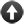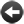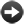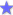§13.14. Relations as values in their own right

As we've seen, most relations have names - "containment relation", for instance. These are themselves values in Inform, though there are a few restrictions on how they are used. (Relations can contain a colossal amount of data, so we don't want to have to copy them casually.)

Consider these two examples:

Parity relates a number (called N) to a number (called M) when N minus M is even.

Joint magnitude relates a number (called N) to a number (called M) when N plus M is greater than 7.

Here "parity relation" and "joint magnitude relation" are both values of the same kind: "relation of numbers to numbers". In general, every relation is a value of kind "relation of K to L", for the appropriate kinds K and L. So the parity relation doesn't have the same kind as the containment relation, for example. Because it often happens that K and L are the same, we can just say "relation of K" in this case, so we could equally say that the kind of the parity relation is "relation of numbers".

This is useful to know when writing phrases like so:

To chart (R - a relation of numbers):
repeat with N running from 1 to 5:
repeat with M running from 1 to 5:
if R relates N to M, say "[N] <=> [M] ";
say "[line break]";

and now "chart parity relation" will work nicely, but "chart visibility relation" will be rejected (as it should be, because it relates things, not numbers). In general, if R is any relation, we can write

if R relates X to Y, ...
now R relates X to Y;
now R does not relate X to Y;

to test, set and unset a relation R between two values. (Inform checks that the values X and Y have the right kind and produces a problem message if not.)

Several useful adjectives can be applied to relations:

"empty" - nothing relates to anything else
"symmetric" - by definition X relates to Y if and only if Y relates to X
"equivalence" - this is a relation "in groups", or an "equivalence relation"
"one-to-one" - it relates one K to one L
"one-to-various" - similarly
"various-to-one" - similarly
"various-to-various" - similarly

So for example it's possible to ask

if R is a symmetric one-to-one relation of texts, ...

With some relations, it's possible to clear them out by writing:

now R is empty;

and with temporary relations (see the next section), it's even possible to change their valencies (one-to-one vs. one-to-various, etc.) using "now", but only when they are empty. The exceptions where "empty" can't be used are those which can't be changed at all, like the parity relation above, and a few built-in cases such as the support, containment and incorporation relations, where emptying would dissolve the model world in a disastrous way.Start of Chapter 13: RelationsBack to §13.13. Relations involving valuesOnward to §13.15. Temporary relationsExampleNumber Study The parity and joint magnitude relations explored.